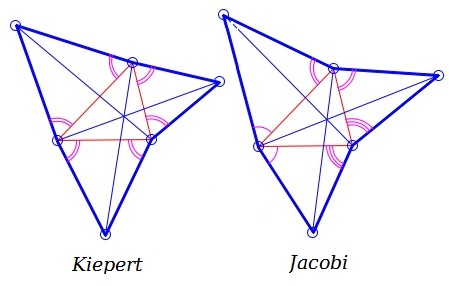# Kiepert's And Jacobi's Theorems What Are They? A Mathematical Droodle

Created with GeoGebra

ExplanationOne of the consequences of Napoleon's Theorem is the existence of Fermat's point. The latter is the point of concurrency of the three lines, each joining a vertex of the given triangle with the farthest vertex of the opposite Napoleon triangle.

Ludwig Kiepert showed that concurrency takes place when equilateral triangles are replaced with similar isosceles triangles. Karl Friedrich Andreas Jacobi generalized even further by proving the presence of concurrency in case the add-on triangles share angles pairwise at each of he base triangle vertices.Kiepert's and Jacobi's Theorems admit a further generalization where the bases of the isosceles triangles need not coincide with the entire sides of the given triangle.

### References

1. J. Baker, Napoleon's Theorem and Beyond, Spreadsheets in Education, v. 1, n. 2.
2. D. Gale, Tracking The Autmatic Ant, Springer-Verlag, 1998### Napoleon's Theorem